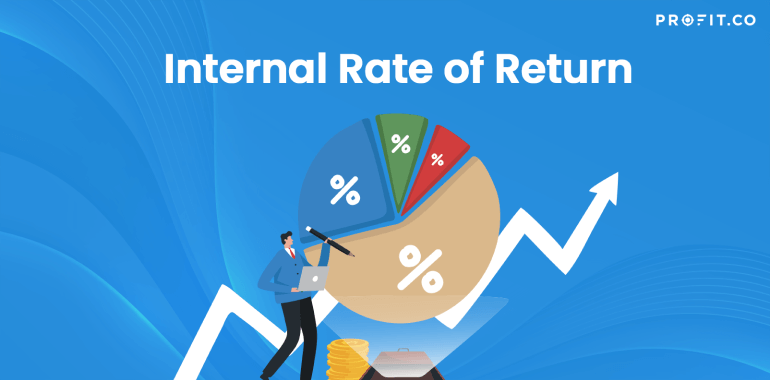Category: Finance KPIs.

The internal rate of return, also abbreviated as IRR, is used to identify which future projects or capital investments will have an acceptable return – it is represented by a minimum discount rate.

Basically, the internal rate of return is a rate that’s equal to the net present value of the future cash flows of a certain project, up until it reaches zero. For example, if we are to calculate the present value of the future cash flows using the project’s internal rate as its discount rate and then subtract the original investment, the net present value of a certain project would be equal to zero.

The Basics of Internal Rate of Return

While it may seem confusing, things are about to get a lot simpler. We have to think of this as capital investing. When the management team of a company is about to invest capital, they have to calculate the percentage return.

However, this is not everything. They also need to know the exact percentage return that it’s required to eventually break even on an investment, while it is adjusted for the time value of money.

The internal rate of return is basically the interest percentage that a company has and wants to achieve in order to be equal in terms of its investment in new capital.

Naturally, a company thinks of breaking even on a certain investment as, probably, the worst-case scenario – as they consider it the minimum acceptable return on an investment. Of course, profit is sought after.

What is its Formula?

So, the formula is quite complex – complex as in long; it’s still very easy to round up the internal rate of return.

Our main goal, when calculating the internal rate of return, is to make equal the sum of the present value of future cash flow, from which we subtract the initial investment, with zero.

Therefore, we will have the cash flows for certain years which will be separately divided by (1+IRR), after which the initial investment will be subtracted from the result.

Naturally, the IRR is the only variable in the equation that the management team of a company won’t know. This means that they will have to estimate the future income of the investment, based on the capital that is required to start the project.

By doing so, they will have the discount rate that makes the NPV be equal to zero.

Final Thoughts

So, the internal rate of return is basically a rate at which the net present value of the costs equals the net present value of the expected future revenue of a certain investment.

Companies usually calculate the internal rate of return for a number of projects they want to invest their capital in – therefore, it is clear that they will decide to invest in something that is profitable in the future and will pass on the poor investments.

Of course, since the companies are working with expected values, they can’t always be sure of their investment, but calculating the internal rate of return gives them some time and insight in regard to their investments and purchases.

## Related Articles

• ### Sharpe Ratio

In order to be sound in your financial goals, you need to have a great source of knowledge on every... Read more